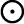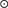HomeNAAP LabsEclipsing Binary Stars › Luminosity

# The Luminosity of Eclipsing Binary Systems

Luminosity is the total energy that a star produces in one second. It depends on both the radius of the star and on its surface temperature. One can calculate luminosity by finding the product of 1) how much energy each section of the surface of a star is producing (σT4, the Stefan-Boltzmann Law) and 2) the entire surface area of the star (R2). Thus, the luminosity of a star (σT4R2) would increase if one increased either the size R or the surface temperature T with temperature being the dominating factor. If we work in solar units indicated with asymbol, we can ignore the constants and simply write L = R2T4.

For example, if a star has the same surface temperature as the sun and a radius that is twice as big, its luminosity must be L = (2 R)2(T)4 = 4L.

One can experiment with the relationships between luminosity, temperature (spectral type), and radius with the Stellar Luminosity Calculator. Use the calculator to answer the following questions.

 What is the luminosity of a star that is the twice as big as the sun but has the same surface temperature? What is the luminosity of a star that is half as big as the sun and twice as hot? Estimate the size of a star that has the same surface temperature as the sun but produces 100 times as much energy.

The maximum luminosity of a binary system consists of the combined luminosities of the two stars. However, when one star eclipses another along our line of sight the total luminosity falls. The drop in the luminosity depend upon many factors including the area of the more distant star that is obscured. Since the cross-sectional area of a star is the area of a circle whose radius is the star's radius — the problem is simply one of intersecting circles. The animation to the right allows you to play with intersecting circles. Experiment with it to develop some intuition related to intersecting circles.

• Use the white circle to obscure 50% of the yellow circle.
• Use the yellow circle to obscure 80% of the white circle.

The situation with actual stars is far more complicated since the two stars are typically not the same size nor the same surface temperature. One can experiment with stellar eclipse with stars of different sizes and different temperatures with the simulator below.

 First experiment with the two settings where the stars have the same temperature. Verbalize a rule relating the area obscured to the drop in total luminosity indicated by the Light Meter? Click on the "Same Size, Different Temperature" setting. Is the luminosity drop when the blue star completely obscures the orange star the same as when the orange star eclipses the blue star? Next experiment with the "Different Size, Different Temperature" setting. Is the Light Meter sufficiently sensitive to detect when the cooler star is completely eclipsed?